SNAP Library 6.0, Developer Reference  2020-12-09 16:24:20 SNAP, a general purpose, high performance system for analysis and manipulation of large networks
TSnap::TPRManager Class Reference

Push relabel attr manager. More...

Collaboration diagram for TSnap::TPRManager: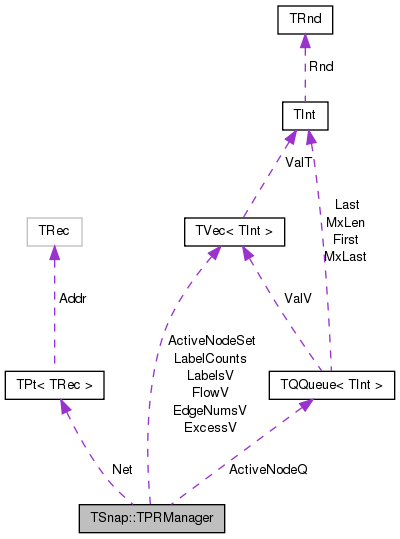[legend]

## Public Member Functions

TPRManager (PNEANet &Net)

int Capacity (int EId)

int & Flow (int EId)

int Label (int NId)

int & Excess (int NId)

int & EdgeNum (int NId)

void SetLabel (int NId, int Label)

void CheckGap (int GapLabel)
Removes any gaps in node labels. More...

bool HasActive ()

int IsActive (int NId)

int PopActive ()

void PushActive (int NId)

void RemoveActive (int NId)

int GetMaxLabel ()

## Private Attributes

PNEANetNet

int CapIndex

TIntV FlowV

TIntV ExcessV

TIntV EdgeNumsV

TIntV LabelsV

TIntV LabelCounts

int LabelLimit

int MaxLabel

TIntQ ActiveNodeQ

TIntV ActiveNodeSet

int ActiveCount

## Detailed Description

Push relabel attr manager.

Flow algorithms require 2 attributes to be defined on each edge: Capacity: The maximum amount of flow over the edge. Flow: The current amount of flow over the edge. Push Relabel requires 3 attributes to be defined on each node: Label: An estimate of the distance from this node to the sink. Push Relabel pushes flow from nodes of higher labels to those of lower labels. Excess: Sum of flows into the node minus sum of flows leaving the nodes. Push Relabel finds nodes with positive excess and pushes flow out of them. These values will never be negative. EdgeNum: Push Relabel needs to cycle through the edges of a node; this attribute keeps track of which edge the cycle is at. The TPRManager class keeps maintains all of these attributes over the course of the algorithm, mostly to make the code easier to understand. It also maintains a queue of active nodes, which are nodes with positive excess and label < N, where N is the number of nodes. Each iteration of the Push Relabel algorithm pops an active node form the queue and tries to push flow from it. The current implementation of the active queue is a FIFO queue. A highest label first scheme for the active queue is also very common.

Definition at line 167 of file flow.cpp.

## Constructor & Destructor Documentation

 TSnap::TPRManager::TPRManager ( PNEANet & Net )
inline

Definition at line 169 of file flow.cpp.

References ActiveNodeSet, Capacity(), TSnap::CapAttrName, CapIndex, EdgeNumsV, ExcessV, FlowV, IAssert, and LabelCounts.

169  : Net(Net), CapIndex(0), FlowV(Net->GetMxEId()), ExcessV(Net->GetMxNId()), EdgeNumsV(Net->GetMxNId()), LabelsV(Net->GetMxNId()), LabelCounts(Net->GetNodes() + 1), LabelLimit(0), MaxLabel(Net->GetNodes()), ActiveNodeSet(Net->GetMxNId()), ActiveCount(0) {
170  CapIndex = Net->GetIntAttrIndE(CapAttrName);
171  for (int i = 0; i <= Net->GetNodes(); i++) { LabelCounts[i] = 0; }
172  for (TNEANet::TEdgeI EI = Net->BegEI(); EI != Net->EndEI(); EI++) {
173  int EId = EI.GetId();
174  IAssert(Capacity(EId) >= 0);
175  FlowV[EId] = 0;
176  }
177  for (TNEANet::TNodeI NI = Net->BegNI(); NI != Net->EndNI(); NI++) {
178  int NId = NI.GetId();
179  ExcessV[NId] = 0;
180  EdgeNumsV[NId] = 0;
181  ActiveNodeSet[NId] = 0;
182  }
183  LabelCounts = Net->GetNodes();
184  }
#define IAssert(Cond)
Definition: bd.h:262
const TStr CapAttrName
Definition: flow.h:4
Node iterator. Only forward iteration (operator++) is supported.
Definition: network.h:1792
TIntV EdgeNumsV
Definition: flow.cpp:276
TIntV ActiveNodeSet
Definition: flow.cpp:284
int Capacity(int EId)
Definition: flow.cpp:186
TIntV LabelCounts
Definition: flow.cpp:279
Edge iterator. Only forward iteration (operator++) is supported.
Definition: network.h:1867
PNEANet & Net
Definition: flow.cpp:271

Here is the call graph for this function: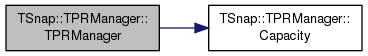## Member Function Documentation

 int TSnap::TPRManager::Capacity ( int EId )
inline

Definition at line 186 of file flow.cpp.

References CapIndex, and Net.

186  {
187  return Net->GetIntAttrIndDatE(EId, CapIndex);
188  }
PNEANet & Net
Definition: flow.cpp:271

Here is the caller graph for this function: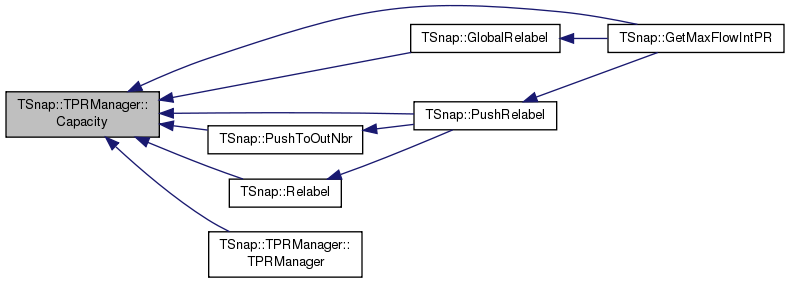void TSnap::TPRManager::CheckGap ( int GapLabel )
inline

Removes any gaps in node labels.

This method implements the gap heuristic. During the algorithm, if some label L no longer has any nodes of that label, but there are nodes with labels K > L, then all of these nodes with labels K > L will not be able to push. Mark these nodes to no longer participate in pushing flow by setting their label to N, where N is the number of nodes.

Definition at line 221 of file flow.cpp.

References IsActive(), LabelCounts, LabelLimit, LabelsV, MaxLabel, Net, and RemoveActive().

Referenced by SetLabel().

221  {
222  for (TNEANet::TNodeI NI = Net->BegNI(); NI != Net->EndNI(); NI++) {
223  int NId = NI.GetId();
224  int OldLabel = LabelsV[NId];
225  if (OldLabel > GapLabel && OldLabel <= LabelLimit) {
226  LabelsV[NId] = MaxLabel;
227  LabelCounts[OldLabel]--;
229  if (IsActive(NId)) { RemoveActive(NId); }
230  }
231  }
232  LabelLimit = GapLabel - 1;
233  }
Node iterator. Only forward iteration (operator++) is supported.
Definition: network.h:1792
int IsActive(int NId)
Definition: flow.cpp:239
void RemoveActive(int NId)
Definition: flow.cpp:263
TIntV LabelCounts
Definition: flow.cpp:279
PNEANet & Net
Definition: flow.cpp:271

Here is the call graph for this function: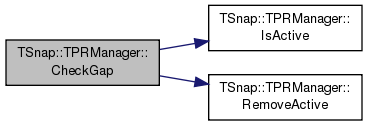Here is the caller graph for this function: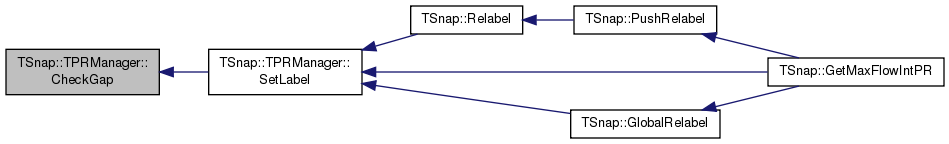int& TSnap::TPRManager::EdgeNum ( int NId )
inline

Definition at line 202 of file flow.cpp.

References EdgeNumsV.

Referenced by TSnap::PushRelabel().

202  {
203  return EdgeNumsV[NId].Val;
204  }
TIntV EdgeNumsV
Definition: flow.cpp:276

Here is the caller graph for this function: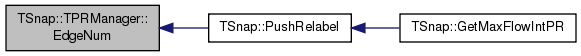int& TSnap::TPRManager::Excess ( int NId )
inline

Definition at line 198 of file flow.cpp.

References ExcessV.

198  {
199  return ExcessV[NId].Val;
200  }

Here is the caller graph for this function: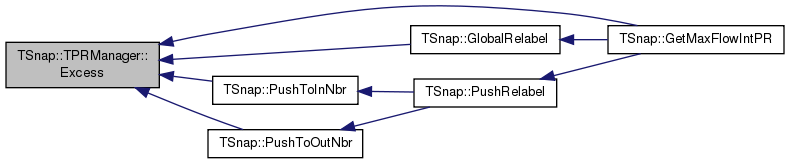int& TSnap::TPRManager::Flow ( int EId )
inline

Definition at line 190 of file flow.cpp.

References FlowV.

190  {
191  return FlowV[EId].Val;
192  }

Here is the caller graph for this function: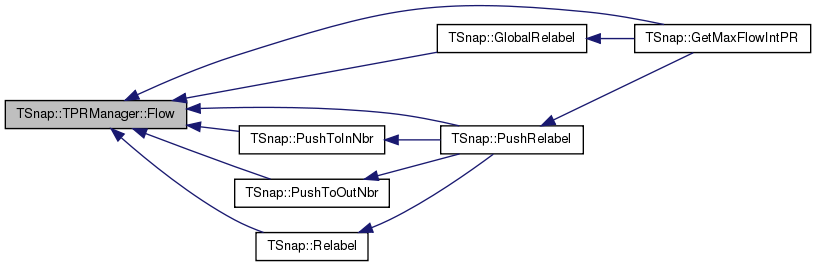int TSnap::TPRManager::GetMaxLabel ( )
inline

Definition at line 268 of file flow.cpp.

References MaxLabel.

Referenced by TSnap::GetMaxFlowIntPR(), TSnap::GlobalRelabel(), and TSnap::Relabel().

268 { return MaxLabel; }

Here is the caller graph for this function: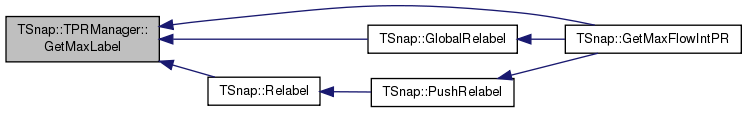bool TSnap::TPRManager::HasActive ( )
inline

Definition at line 235 of file flow.cpp.

References ActiveCount.

Referenced by TSnap::GetMaxFlowIntPR().

235  {
236  return ActiveCount > 0;
237  }

Here is the caller graph for this function: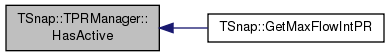int TSnap::TPRManager::IsActive ( int NId )
inline

Definition at line 239 of file flow.cpp.

References ActiveNodeSet.

Referenced by CheckGap(), TSnap::GetMaxFlowIntPR(), and TSnap::GlobalRelabel().

239  {
240  return ActiveNodeSet[NId];
241  }
TIntV ActiveNodeSet
Definition: flow.cpp:284

Here is the caller graph for this function: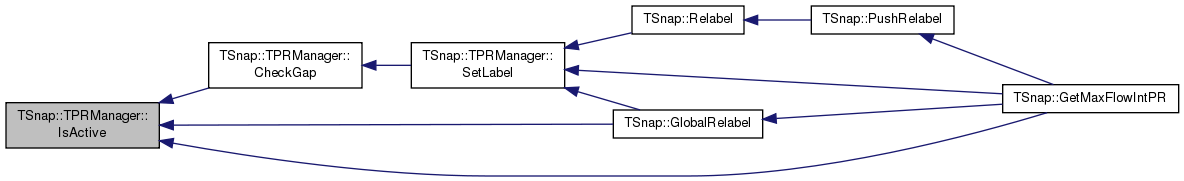int TSnap::TPRManager::Label ( int NId )
inline

Definition at line 194 of file flow.cpp.

References LabelsV.

194  {
195  return LabelsV[NId];
196  }

Here is the caller graph for this function: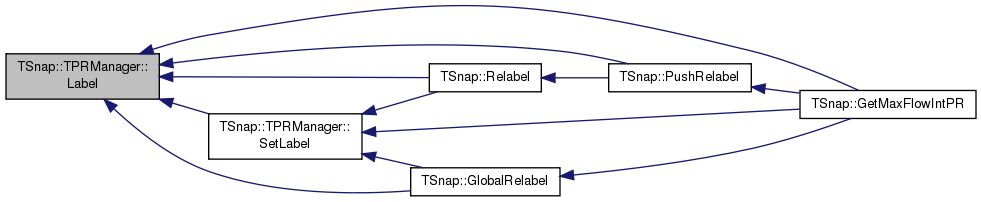int TSnap::TPRManager::PopActive ( )
inline

Definition at line 243 of file flow.cpp.

Referenced by TSnap::GetMaxFlowIntPR().

243  {
244  while (true) {
246  int NId = ActiveNodeQ.Top();
247  ActiveNodeQ.Pop();
248  if (ActiveNodeSet[NId]) {
249  ActiveNodeSet[NId] = 0;
250  ActiveCount--;
251  return NId;
252  }
253  }
254  return -1;
255  }
#define IAssert(Cond)
Definition: bd.h:262
bool Empty() const
Definition: ds.h:2646
void Pop()
Definition: ds.h:2650
TIntQ ActiveNodeQ
Definition: flow.cpp:283
TIntV ActiveNodeSet
Definition: flow.cpp:284
const TVal & Top() const
Definition: ds.h:2648

Here is the call graph for this function: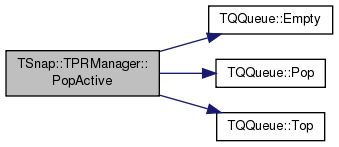Here is the caller graph for this function: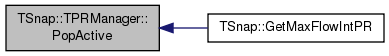void TSnap::TPRManager::PushActive ( int NId )
inline

Definition at line 257 of file flow.cpp.

References ActiveCount, ActiveNodeQ, ActiveNodeSet, and TQQueue< TVal >::Push().

Referenced by TSnap::GetMaxFlowIntPR(), and TSnap::GlobalRelabel().

257  {
258  ActiveNodeSet[NId] = 1;
259  ActiveNodeQ.Push(NId);
260  ActiveCount++;
261  }
TIntQ ActiveNodeQ
Definition: flow.cpp:283
TIntV ActiveNodeSet
Definition: flow.cpp:284
void Push(const TVal &Val)
Definition: ds.h:2653

Here is the call graph for this function: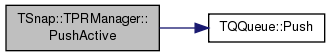Here is the caller graph for this function:void TSnap::TPRManager::RemoveActive ( int NId )
inline

Definition at line 263 of file flow.cpp.

References ActiveCount, and ActiveNodeSet.

Referenced by CheckGap(), and TSnap::GlobalRelabel().

263  {
264  ActiveNodeSet[NId] = 0;
265  ActiveCount--;
266  }
TIntV ActiveNodeSet
Definition: flow.cpp:284

Here is the caller graph for this function: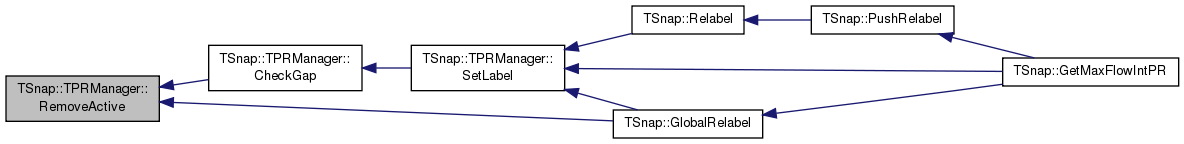void TSnap::TPRManager::SetLabel ( int NId, int Label )
inline

Definition at line 206 of file flow.cpp.

References CheckGap(), Label(), LabelCounts, LabelLimit, LabelsV, MAX, and MaxLabel.

Referenced by TSnap::GetMaxFlowIntPR(), TSnap::GlobalRelabel(), and TSnap::Relabel().

206  {
207  if (Label > LabelLimit + 1) { Label = MaxLabel; }
208  int OldLabel = LabelsV[NId];
209  LabelsV[NId] = Label;
210  LabelCounts[OldLabel]--;
211  LabelCounts[Label]++;
212  if (Label != MaxLabel) { LabelLimit = MAX(LabelLimit, Label); }
213  if (LabelCounts[OldLabel] == 0) { CheckGap (OldLabel); }
214  }
void CheckGap(int GapLabel)
Removes any gaps in node labels.
Definition: flow.cpp:221
int Label(int NId)
Definition: flow.cpp:194
TIntV LabelCounts
Definition: flow.cpp:279
#define MAX(a, b)
Definition: bd.h:350

Here is the call graph for this function: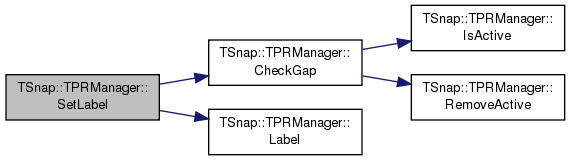Here is the caller graph for this function: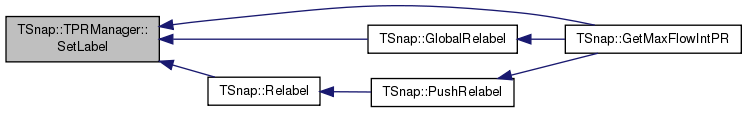## Member Data Documentation

 int TSnap::TPRManager::ActiveCount
private

Definition at line 285 of file flow.cpp.

Referenced by HasActive(), PopActive(), PushActive(), and RemoveActive().

 TIntQ TSnap::TPRManager::ActiveNodeQ
private

Definition at line 283 of file flow.cpp.

Referenced by PopActive(), and PushActive().

 TIntV TSnap::TPRManager::ActiveNodeSet
private

Definition at line 284 of file flow.cpp.

Referenced by IsActive(), PopActive(), PushActive(), RemoveActive(), and TPRManager().

 int TSnap::TPRManager::CapIndex
private

Definition at line 272 of file flow.cpp.

Referenced by Capacity(), and TPRManager().

 TIntV TSnap::TPRManager::EdgeNumsV
private

Definition at line 276 of file flow.cpp.

Referenced by EdgeNum(), and TPRManager().

 TIntV TSnap::TPRManager::ExcessV
private

Definition at line 275 of file flow.cpp.

Referenced by Excess(), and TPRManager().

 TIntV TSnap::TPRManager::FlowV
private

Definition at line 273 of file flow.cpp.

Referenced by Flow(), and TPRManager().

 TIntV TSnap::TPRManager::LabelCounts
private

Definition at line 279 of file flow.cpp.

Referenced by CheckGap(), SetLabel(), and TPRManager().

 int TSnap::TPRManager::LabelLimit
private

Definition at line 280 of file flow.cpp.

Referenced by CheckGap(), and SetLabel().

 TIntV TSnap::TPRManager::LabelsV
private

Definition at line 278 of file flow.cpp.

Referenced by CheckGap(), Label(), and SetLabel().

 int TSnap::TPRManager::MaxLabel
private

Definition at line 281 of file flow.cpp.

Referenced by CheckGap(), GetMaxLabel(), and SetLabel().

 PNEANet& TSnap::TPRManager::Net
private

Definition at line 271 of file flow.cpp.

Referenced by Capacity(), and CheckGap().

The documentation for this class was generated from the following file: